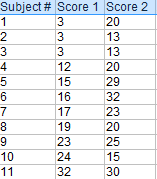# Comparison of Means

Inferential Statistics > Comparison of Means

## Comparison of Means TechniquesComparison of means tests helps you determine if your groups have similar means.There are many cases in statistics where you’ll want to compare means for two populations or samples. Which technique you use depends on what type of data you have and how that data is grouped together.

The four major ways of comparing means from data that is assumed to be normally distributed are:

1. Independent Samples T-Test. Use the independent samples t-test when you want to compare means for two data sets that are independent from each other. Click here for a step by step article.
2. One sample T-Test. Choose this when you want to compare means between one data set and a specified constant (like the mean from a hypothetical normal distribution). Click here for a step by step article.
3. Paired Samples T-Test. Use this test if you have one group tested at two different times. In other words, you have two measurements on the same item, person, or thing.The groups are “paired” because there intrinsic connections between them (i.e. they are not independent). This comparison of means is often used for groups of patients before treatment and after treatment, or for students tested before remediation and after remediation. Click here for a step by step article.
4. One way Analysis of Variance (ANOVA). Although not really a test for comparison of means, ANOVA is the main option when you have more than two levels of independent variable. For example, if your independent variable was “brand of coffee” your levels might be Starbucks, Peets and Trader Joe’s. Use this test when you have a group of individuals randomly split into smaller groups and completing different tasks (like drinking different coffee).

## Non Normal Data

If you have non-normal data (or if you don’t know what distribution your data comes from), you can’t use any of the above tests for comparison of means. You must use a non-parametric test (non-parametric basically means that you don’t know the distribution’s parameters):

1. For independent samples, use the Mann-Whitney U test. This test is essentially the same as the t-test for independent samples.
2. For paired groups, use Wilcoxon Signed Rank. This test compares medians, not means.

## References

Beyer, W. H. CRC Standard Mathematical Tables, 31st ed. Boca Raton, FL: CRC Press, pp. 536 and 571, 2002.
Everitt, B. S.; Skrondal, A. (2010), The Cambridge Dictionary of Statistics, Cambridge University Press.
Vogt, W.P. (2005). Dictionary of Statistics & Methodology: A Nontechnical Guide for the Social Sciences. SAGE.
Wheelan, C. (2014). Naked Statistics. W. W. Norton & Company

CITE THIS AS:
Stephanie Glen. "Comparison of Means" From StatisticsHowTo.com: Elementary Statistics for the rest of us! https://www.statisticshowto.com/comparison-of-means/
---------------------------------------------------------------------------Need help with a homework or test question? With Chegg Study, you can get step-by-step solutions to your questions from an expert in the field. Your first 30 minutes with a Chegg tutor is free!